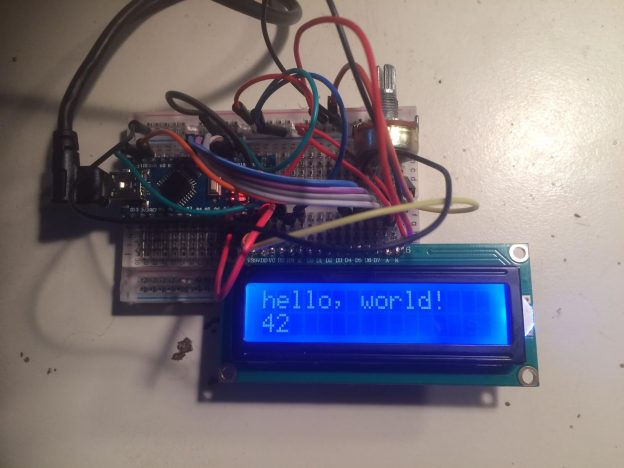# LiquidCrystal-Display blue 1602 Arduino Nano

I recently bought several “Smart Electronics LCD Module Display Monitor 1602 5V Blue” for building a wifi alarm clock. It uses the very common Hitachi HD44780 driver. To verify if they are working I followed this well described tutorial on arduino.cc and used a arduino nano for that. Removing the 10k ohms resistor, because it dimmed the LED background light way to much and after adjusting the potentiometer to about 1,64k ohms the visibility of desired output was optimal.

For the sake of documentation the:

## Wiring

``` LCD    -> Arduino
--------------------------
1|VSS| -> Arduino GND
2|VDD| -> Arduino +5v
3|VO | -> Arduino GND pin + 1,6k ohm Resistor or Potentiometer
4|RS | -> Arduino pin 12
5|RW | -> Arduino GND - pin can be conected to 11. But Ground was used here.
6|E  | -> Arduino pin 11
11|D4 | -> Arduino pin 5
12|D5 | -> Arduino pin 4
13|D6 | -> Arduino pin 3
14|D7 | -> Arduino pin 2
15|A  | -> GND + no or a below 10k Resistor  to dimm background light (Backlight power)
16|K  | -> Arduino GND (Backlight ground)```

## Code

```#include <LiquidCrystal.h>

// initialize the library with the numbers of the interface pins
LiquidCrystal lcd(12, 11, 5, 4, 3, 2);

void setup() {
lcd.display();
// set up the LCD's number of columns and rows:
lcd.begin(16, 2);

lcd.print("hello, world!");
}

void loop() {
// set the cursor to column 0, line 1 (second row)
lcd.setCursor(0, 1);
// print the number of seconds since reset:
lcd.print(millis() / 1000);
}```

The next step is to connect it to an esp8266 and figuring out if the new 3.3V signal strength will be enough. A possible solution would be to use the esp8266 only as a communication device.